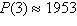Name:    Chapter 5 Pre Test

1.

Select the graph of the function.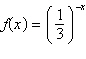a.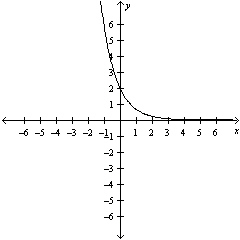d.b.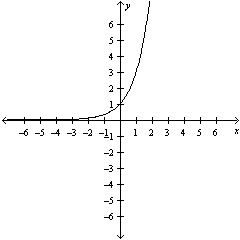e.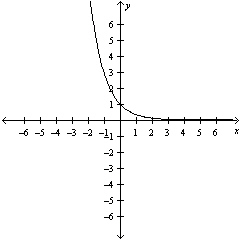c.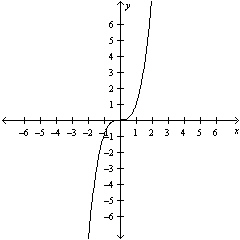2.

Use a graphing utility to construct a table of values for the function. Round your answer to three decimal places.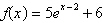a.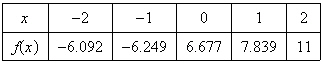b.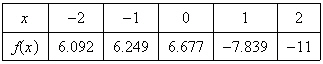c.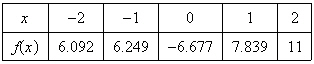d.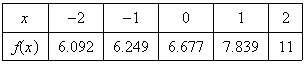e.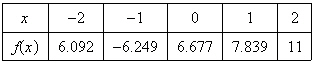3.

Select the graph of the exponential function.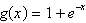a.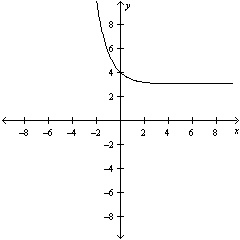d.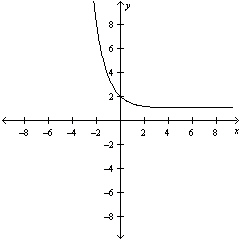b.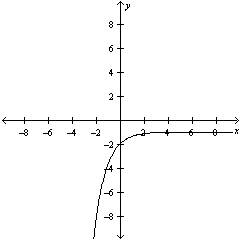e.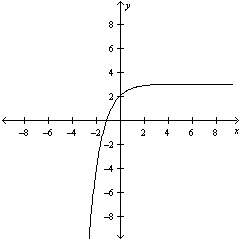c.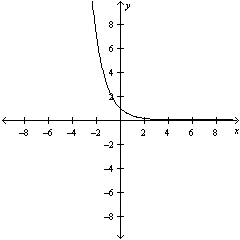4.

Write the logarithmic equation in exponential form.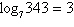a.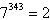b.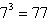c.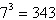d.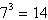e.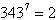5.

Evaluate the function at the indicated value of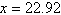. Round your result to three decimal places.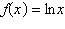a. 3.132 b. –2.033 c. –3.132 d. 2.033 e. 22.92

6.

Evaluate the function at the indicated value of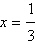. Round your result to three decimal places.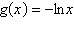a. –1.099 b. 3 c. 1.792 d. –1.792 e. 1.099

7.

Find the exact value of the logarithmic expression without using a calculator.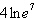a.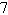b.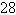c.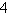d.e.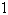8.

Condense the expression to the logarithm of a single quantity.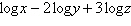a.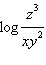b.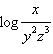c.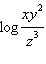d.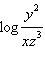e.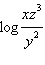9.

Use the properties of logarithms to rewrite and simplify the logarithmic expression.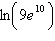a.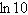b.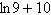c.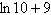d.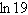e.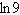10.

Evaluate the logarithm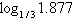using the change of base formula. Round to 3 decimal places.
 a. –1.745 b. –0.692 c. 0.630 d. 0.273 e. –0.573

11.

Find the exact value of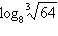without using a calculator.
 a.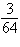b.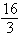c.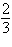d. –1 e.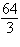12.

Find the exact value of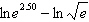without using a calculator.
 a. 5 b. 2.5 c. 1.25 d. 2 e. 3

13.

Solve the exponential equation algebraically. Approximate the result to three decimal places.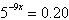a.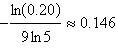b.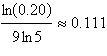c.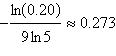d.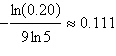e.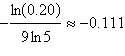14.

Solve the exponential equation algebraically. Approximate the result to three decimal places.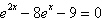a.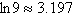b.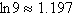c.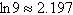d.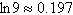e.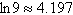15.

Solve the logarithmic equation algebraically. Approximate the result to three decimal places.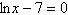a.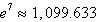b.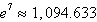c.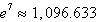d.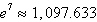e.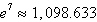16.

Solve the logarithmic equation algebraically. Approximate the result to three decimal places.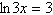a.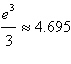b.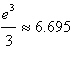c.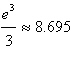d.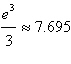e.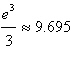17.

Solve the logarithmic equation algebraically. Approximate the result to three decimal places.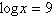a. 100,000,000 b. 100,000,000,000 c. 1,000,000,000 d. 10,000,000,000 e. 10,000,000

18.

Solve the logarithmic equation algebraically. Approximate the result to three decimal places.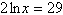a.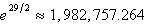b.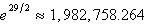c.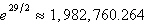d.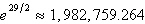e.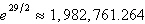19.

Solve the equation algebraically. Round the result to three decimal places.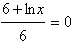a.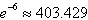b.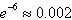c.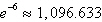d.e.20.

The population P of a culture of bacteria is described by the equation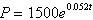, where t is the time, in hours, relative to the time at which the population was 1500. What was the population at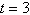hours?
 a.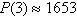b.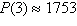c.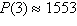d.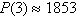e.